# summary-function

Performs statistical summary calculations.

 Restriction: A summary function cannot appear in an ON clause or a WHERE clause. See: GROUP BY Clause HAVING Clause SELECT Clause table-expression Examples: Creating a View from a Query's Result Joining Two Tables and Calculating a New Value Counting Missing Values with a SAS Macro

## Syntax

summary-function (<DISTINCT | ALL> sql-expression)

### Required Arguments

#### summary-function

is one of the following:

#### AVG|MEAN

arithmetic mean or average of values

#### COUNT|FREQ|N

number of nonmissing values

#### CSS

corrected sum of squares

#### CV

coefficient of variation (percent)

largest value

smallest value

#### NMISS

number of missing values

#### PRT

is the two-tailed p-value for Student's t statistic, T with n – 1 degrees of freedom.

range of values

#### STD

standard deviation

#### STDERR

standard error of the mean

sum of values

#### SUMWGT

sum of the WEIGHT variable values

#### T

Student's t value for testing the hypothesis that the population mean is zero

#### USS

uncorrected sum of squares

#### VAR

variance

For a description and the formulas used for these statistics, see “SAS Elementary Statistics Procedures” in Chapter 1 of Base SAS Procedures Guide.

#### DISTINCT

specifies that only the unique values of an SQL expression be used in the calculation.

#### ALL

specifies that all values of an SQL expression be used in the calculation. If neither DISTINCT nor ALL is specified, then ALL is used.

is described in

## Details

### Summarizing Data

Summary functions produce a statistical summary of the entire table or view that is listed in the FROM clause or for each group that is specified in a GROUP BY clause. If GROUP BY is omitted, then all the rows in the table or view are considered to be a single group. These functions reduce all the values in each row or column in a table to one summarizing or aggregate value. For this reason, these functions are often called aggregate functions. For example, the sum (one value) of a column results from the addition of all the values in the column.

### Counting Rows

The COUNT function counts rows. COUNT(*) returns the total number of rows in a group or in a table. If you use a column name as an argument to COUNT, then the result is the total number of rows in a group or in a table that have a nonmissing value for that column. If you want to count the unique values in a column, then specify COUNT(DISTINCT column).
If the SELECT clause of a table expression contains one or more summary functions and that table expression resolves to no rows, then the summary function results are missing values. The following are exceptions that return zeros:
• COUNT(*)
• COUNT(<DISTINCT> sql-expression)
• NMISS(<DISTINCT> sql-expression)

### Calculating Statistics Based on the Number of Arguments

The number of arguments that is specified in a summary function affects how the calculation is performed. If you specify a single argument, then the values in the column are calculated. If you specify multiple arguments, then the arguments or columns that are listed are calculated for each row.
Note: When more than one argument is used within an SQL aggregate function, the function is no longer considered to be an SQL aggregate or summary function. If there is a like-named Base SAS function, then PROC SQL executes the Base SAS function, and the results that are returned are based on the values for the current row. If no like-named Base SAS function exists, then an error will occur. For example, if you use multiple arguments for the AVG function, an error will occur because there is no AVG function for Base SAS.
For example, consider calculations on the following table.
```data summary;
input X Y Z;
datalines;
1 3 4
2 4 5
8 9 4
4 5 4
;```
```proc sql;
title 'Summary Table';
select * from summary;```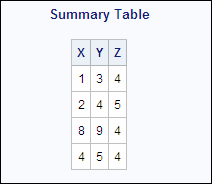If you use one argument in the function, then the calculation is performed on that column only. If you use more than one argument, then the calculation is performed on each row of the specified columns. In the following PROC SQL step, the MIN and MAX functions return the minimum and maximum of the columns that they are used with. The SUM function returns the sum of each row of the columns specified as arguments:
```proc sql;
select min(x) as Colmin_x,
min(y) as Colmin_y,
max(z) as Colmax_z,
sum(x,y,z) as Rowsum
from summary;```
Summary Functions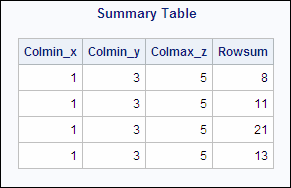### Remerging Data

When you use a summary function in a SELECT clause or a HAVING clause, you might see the following message in the SAS log:
```NOTE: The query requires remerging summary
statistics back with the original
data.```
The process of remerging involves two passes through the data. On the first pass, PROC SQL
• calculates and returns the value of summary functions. It then uses the result to calculate the arithmetic expressions in which the summary function participates.
• groups data according to the GROUP BY clause.
On the second pass, PROC SQL retrieves any additional columns and rows that it needs to show in the output.
Note: To specify that PROC SQL not process queries that use remerging of data, use either the PROC SQL NOREMERGE option or the NOSQLREMERGE system option. If remerging is attempted when the NOMERGE option or the NOSQLREMERGE system option is set, an error is written to the SAS log. For more information, see the and the
The following examples use the PROCLIB.PAYROLL table (shown in ) to show when remerging of data is and is not necessary.
The first query requires remerging. The first pass through the data groups the data by Jobcode and resolves the AVG function for each group. However, PROC SQL must make a second pass in order to retrieve the values of IdNumber and Salary.
```proc sql outobs=10;
title 'Salary Information';
title2 '(First 10 Rows Only)';
select  IdNumber, Jobcode, Salary,
avg(salary) as AvgSalary
from proclib.payroll
group by jobcode;```
Salary Information That Required Remerging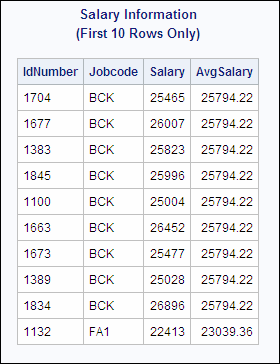You can change the previous query to return only the average salary for each jobcode. The following query does not require remerging because the first pass of the data does the summarizing and the grouping. A second pass is not necessary.
```proc sql outobs=10;
title 'Average Salary for Each Jobcode';
select Jobcode, avg(salary) as AvgSalary
from proclib.payroll
group by jobcode;```
Salary Information That Did Not Require Remerging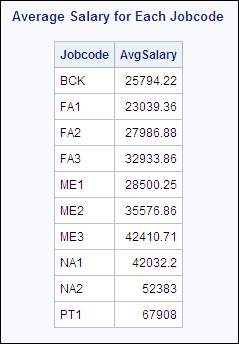When you use the HAVING clause, PROC SQL might have to remerge data to resolve the HAVING expression.
First, consider a query that uses HAVING but that does not require remerging. The query groups the data by values of Jobcode, and the result contains one row for each value of Jobcode and summary information for people in each Jobcode. On the first pass, the summary functions provide values for the `Number`, ```Average Age```, and `Average Salary` columns. The first pass provides everything that PROC SQL needs to resolve the HAVING clause, so no remerging is necessary.
```proc sql outobs=10;
title 'Summary Information for Each Jobcode';
title2 '(First 10 Rows Only)';
select Jobcode,
count(jobcode) as number
label='Number',
avg(int((today()-birth)/365.25))
as avgage format=2.
label='Average Age',
avg(salary) as avgsal format=dollar8.
label='Average Salary'
from proclib.payroll
group by jobcode
having avgage ge 30;```
Jobcode Information That Did Not Require Remerging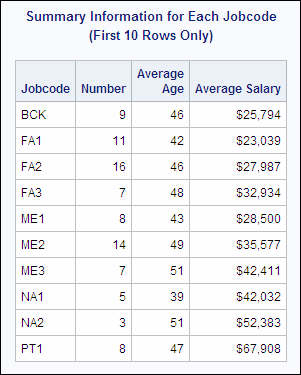In the following query, PROC SQL remerges the data because the HAVING clause uses the SALARY column in the comparison and SALARY is not in the GROUP BY clause.
```proc sql outobs=10;
title 'Employees who Earn More than the';
title2 'Average for Their Jobcode';
title3 '(First 10 Rows Only)';
select Jobcode, Salary,
avg(salary) as AvgSalary
from proclib.payroll
group by jobcode
having salary > AvgSalary;```
Jobcode Information That Did Require Remerging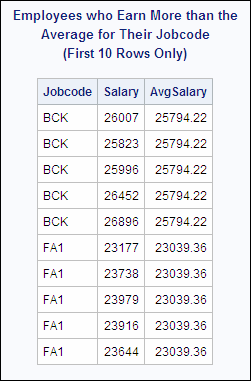Keep in mind that PROC SQL remerges data when
• the values returned by a summary function are used in a calculation. For example, the following query returns the values of X and the percentage of the total for each row. On the first pass, PROC SQL computes the sum of X, and on the second pass PROC SQL computes the percentage of the total for each value of X:
```data summary;
input x;
datalines;
32
86
49
49
;```
```proc sql;
title 'Percentage of the Total';
select X, (100*x/sum(X)) as Pct_Total
from summary;```
```                            Percentage of the Total

x  Pct_Total
-------------------
32   14.81481
86   39.81481
49   22.68519
49   22.68519
```
• the values returned by a summary function are compared to values of a column that is not specified in the GROUP BY clause. For example, the following query uses the PROCLIB.PAYROLL table. PROC SQL remerges data because the column Salary is not specified in the GROUP BY clause:
```proc sql;
select  jobcode,  salary,
avg(salary) as avsal
from proclib.payroll
group by jobcode
having salary > avsal;```
• a column from the input table is specified in the SELECT clause and is not specified in the GROUP BY clause. This rule does not refer to columns used as arguments to summary functions in the SELECT clause.
For example, in the following query, the presence of IdNumber in the SELECT clause causes PROC SQL to remerge the data because IdNumber is not involved in grouping or summarizing during the first pass. In order for PROC SQL to retrieve the values for IdNumber, it must make a second pass through the data.
```proc sql;
select IdNumber, jobcode,
avg(salary) as avsal
from proclib.payroll
group by jobcode;```
FOOTNOTE 1:Currently, there is no way to designate a WEIGHT variable for a table in PROC SQL. Thus, each row (or observation) has a weight of 1.[return]